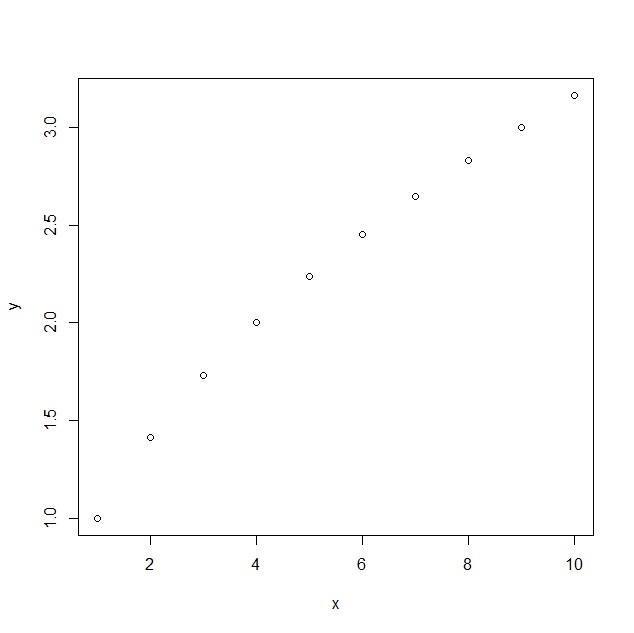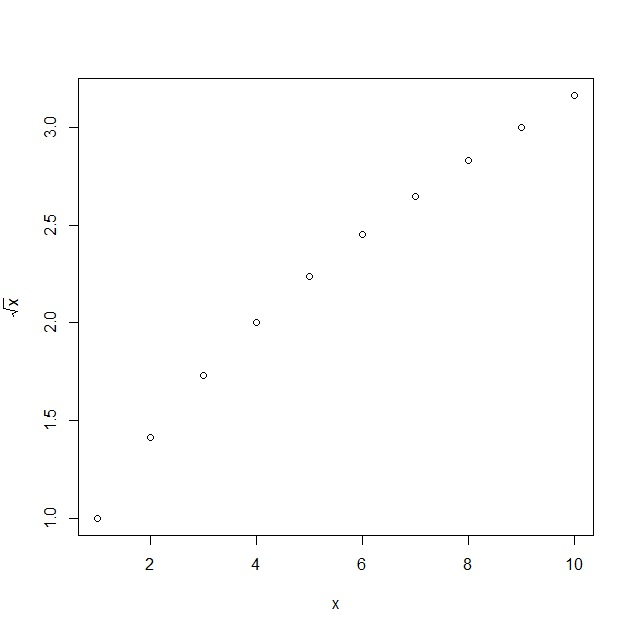• First of all, create a plot having square root values to display default visualisation.

• Then, create the plot with expression function to display square root sign in the plot.

## Example

#### Create plot in base R

Using plot function to create a plot in base R with square root values as shown below −

x<-1:10
y<-sqrt(x)
plot(x,y)

## Outputx<-1:10
plot(x,y,ylab=expression(sqrt(x)))